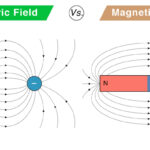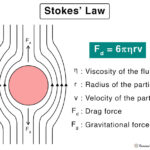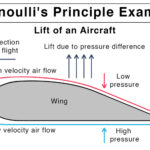Home / Physics / Sliding Friction

# Sliding Friction

## What is Sliding Friction

Sliding friction is considered a resistance to an object’s motion when it slides over a surface. Consider a box lying on a floor. Its surface is in contact with the surface of the floor. When a person pushes the box, it slides over the floor. However, the person has to overcome the resistance offered by the floor. This resistance is known as sliding friction. It is a type of kinetic friction in which two surfaces are in contact and moves relative to one another.

Surfaces are not always perfectly smooth. Friction arises due to tiny bumps and hills that are present on surfaces. As a result, when one surface comes in contact with another, these bumps interlock and hinder the motion. Because there is no liquid between the surfaces, the phenomenon is also called dry sliding friction.

## Examples of Sliding Friction

Here are some examples of the sliding friction that one can find in daily life.

• Rubbing the hands together generates heat due to friction
• Difficulty in pushing a fridge on a floor
• Skidding of a car when trying to brake
• Slowing of motion during sledding and skiing

## Characteristics of Sliding Friction

Here are some facts and properties of the sliding friction force.

• Contact force
• Opposes the motion of an object
• The direction is opposite to the force applied to the object

## Laws of Sliding Friction

Here are some laws of sliding friction.

• Proportional to the normal force or load
• Independent of the area of contact
• Independent of the sliding speed

## How to Calculate Sliding Friction

The sliding friction equation can be calculated by applying physics principles and Newton’s laws of motion.

### Sliding Friction Formula

Suppose a force is acting on an object of mass m that displaces it on a surface. According to Newton’s third law of motion, the surface will apply an equal and opposite force on the object, which is the force of sliding friction FK. According to friction laws, the frictional force is proportional to the normal load FN. Therefore,

FK FL

Or, FK = μK FL

Where μK is known as the coefficient of sliding friction. Its value is a constant depending on the surfaces in contact. It is unitless and dimensionless. The unit of friction is Newton or N.

Again, according to Newton’s third law of motion, the normal force is equal to the object’s weight mg.

FN = mg

Therefore, the magnitude of sliding friction is given by

FK = μK mg

From the above equation, it is clear that the weight influences sliding friction. As the weight increases, the friction increases proportionately.

Now, suppose the object is moving with an acceleration a. According to Newton’s second law, it experiences a force ma given by

ma = FA – FK

ma = FAμK mg

Therefore, the acceleration is given by

a = FA/m – μK g

## How to Reduce Sliding Friction

The sliding friction can be reduced by

• Introducing a lubricant between the two surfaces such that the dry friction is converted into lubrication
• Using rollers, like a ball bearing, that changes sliding friction into rolling friction easily
• Reducing the normal load since the frictional force is proportional to the normal force

## Sliding and Rolling Friction

If an object rolls over a surface instead of sliding, then the resistance to motion is known as rolling friction.

### Why is Sliding Friction More Than Rolling Friction

The cause of sliding friction is the interlocking of the bumps and hills present on surfaces. When the contact area is large, then the friction force will be high. In the case of rolling friction, there is a layer of rollers between two surfaces. As a result, the contact between the flat surfaces and the curved rollers is minimum. Hence, the friction is reduced. Below are some differences between sliding and rolling friction.

### Sliding Friction vs. Rolling Friction

Article was last reviewed on Wednesday, November 25, 2020

### Related articlesElectric Field vs. Magnetic FieldHooke’s LawStokes’ LawBernoulli’s Principle and Equation